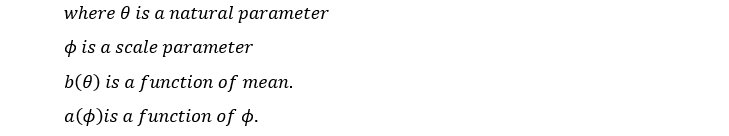Search
• Sneha Khanna

# Generalised Linear Model | GLM

The generalized linear model is used to model data that belongs to a distribution that is a member of an exponential family.

To check, if a distribution belongs to an exponential family, this equation needs to get satisfied:-GLM consists of:

1. A distribution

2. Linear predictor

In GLM, an explanatory variable can be a variable or a factor.

Variable:- It takes real numerical value. For eg: age, weight, etc.

Factor:- It is qualitative. For eg: gender, location, etc.

Written by: Sneha Khanna

Email ID: sneha12khanna@gmail.com